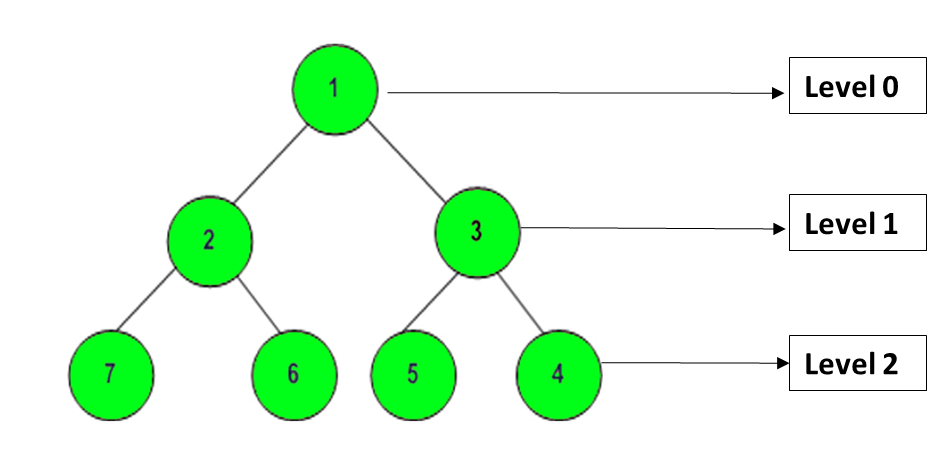Related Articles

# Level order traversal in spiral form using stack and multimap

• Difficulty Level : Hard
• Last Updated : 01 Jul, 2020

Given a binary tree of N nodes, the task is to print level order traversal in a spiral form. In spiral form, nodes at the first and second level of tree are printed normally (left to right), after which nodes at alternate levels are printed in reverse order.

Examples:

Input: N = 3

```      1
/ \
3   2
```

Output: 1 3 2
Explanation:
Nodes at level 0 printed in normal order (1)
Nodes at level 1 printed in normal order (3, 2)
Hence, spiral order is (1, 3, 2)

Input: N = 5

```       10
/  \
20  30
/  \
40  60
```

Output: 10 20 30 60 40
Explanation:
Nodes at level 0 printed in normal order (10)
Nodes at level 1 printed in normal order (20, 30)
Nodes at level 2 printed in reverse order (60, 40)
Hence, spiral order is (10, 20, 30, 60, 40)

## Recommended: Please try your approach on {IDE} first, before moving on to the solution.

Naive Approach:
A naive approach for this problem has already been discussed in this article. The basic idea is to use recursion and a flag variable, using which nodes of alternate levels are printed in reverse order and finally spiral form is obtained.
Time complexity: O(N2)
Auxiliary Space: O(1)

Efficient Approach:
In this approach, stack and multimap are used. A multimap container in C++ stores the (key, value) pairs in ascending order, sorted according to the key. For every node of a given tree, if we put (level, node) in a multimap, then it will store these nodes sorted according to their level.

For example, a given tree is:For this tree, the multimap would look like:

```Key(Level)     Value(Element)
0               1
1               2
1               3
2               7
2               6
2               5
2               4
```

Detailed steps of this approach are as follows:

1. Traverse the given tree and insert all (level, node) pairs in a multimap and then traverse this multimap.
2. If the level is odd, print the nodes in order in which they are present in the multimap.
3. If the level is even, push all elements of the current level to a stack then pop all elements from the stack and print them. It gives the reverse order.

Finally, this level order traversal will result in the required spiral form.

Below is the implementation of the above approach:

 `// C++ implementation of``// the above approach`` ` `#include ``using` `namespace` `std;`` ` `// Tree Node``struct` `Node {``    ``int` `data;``    ``Node* left;``    ``Node* right;``};`` ` `// Utility function to``// create a new Tree Node``Node* newNode(``int` `val)``{``    ``Node* temp = ``new` `Node;``    ``temp->data = val;``    ``temp->left = NULL;``    ``temp->right = NULL;`` ` `    ``return` `temp;``}`` ` `void` `printSpiral(Node* root);`` ` `// Function to build tree``// from given nodes``Node* buildTree(string str)``{``    ``// Corner Case``    ``if` `(str.length() == 0``        ``|| str == ``'N'``)``        ``return` `NULL;`` ` `    ``// Vector to store nodes``    ``// after splitting space``    ``vector ip;`` ` `    ``istringstream iss(str);``    ``for` `(string str; iss >> str;)``        ``ip.push_back(str);`` ` `    ``// Creating root of the tree``    ``Node* root = newNode(stoi(ip));`` ` `    ``// Push the root to the queue``    ``queue queue;``    ``queue.push(root);`` ` `    ``// Start from second element``    ``int` `i = 1;``    ``while` `(!queue.empty()``           ``&& i < ip.size()) {`` ` `        ``// Get and remove the``        ``// front of the queue``        ``Node* currNode = queue.front();``        ``queue.pop();`` ` `        ``// Get the current node's``        ``// value from the string``        ``string currVal = ip[i];`` ` `        ``// If left child is not null``        ``if` `(currVal != ``"N"``) {`` ` `            ``// Create the left child``            ``// for the current node``            ``currNode->left = newNode(stoi(currVal));`` ` `            ``// Push it to the queue``            ``queue.push(currNode->left);``        ``}`` ` `        ``// For the right child``        ``i++;``        ``if` `(i >= ip.size())``            ``break``;``        ``currVal = ip[i];`` ` `        ``// If the right child is not null``        ``if` `(currVal != ``"N"``) {`` ` `            ``// Create the right child``            ``// for the current node``            ``currNode->right = newNode(stoi(currVal));`` ` `            ``// Push it to the queue``            ``queue.push(currNode->right);``        ``}``        ``i++;``    ``}`` ` `    ``return` `root;``}`` ` `// Globally defined multimap``multimap<``int``, ``int``> m;`` ` `// Function to fill multimap``void` `fillMultiMap(Node* root, ``int` `level)``{`` ` `    ``if` `(root == NULL)``        ``return``;`` ` `    ``else` `{``        ``m.insert(pair<``int``, ``int``>(``            ``level, root->data));``        ``fillMultiMap(``            ``root->left, level + 1);``        ``fillMultiMap(``            ``root->right, level + 1);``    ``}``}`` ` `void` `printSpiral(Node* root)``{``    ``m.clear();``    ``fillMultiMap(root, 0);``    ``stack<``int``> s;`` ` `    ``map<``int``, ``int``>::iterator it``        ``= m.begin();`` ` `    ``// Traversing multimap``    ``while` `(it != m.end()) {`` ` `        ``// If level is odd``        ``if` `((it->first) % 2 != 0) {`` ` `            ``// Printing same order``            ``while` `(!s.empty()) {``                ``cout << s.top() << ``" "``;``                ``s.pop();``            ``}``            ``cout << it->second << ``" "``;``        ``}`` ` `        ``// Otherwise, pushing to stack``        ``else` `{``            ``s.push(it->second);``        ``}``        ``it++;``    ``}`` ` `    ``// Pop from stack``    ``// to get reverse order``    ``while` `(!s.empty()) {``        ``cout << s.top() << ``" "``;``        ``s.pop();``    ``}`` ` `    ``return``;``}`` ` `// Driver code``int` `main()``{`` ` `    ``// Tree input``    ``string s = ``"1 2 3 7 6 5 4"``;`` ` `    ``// Build tree form given nodes``    ``Node* root = buildTree(s);`` ` `    ``// Print spiral form``    ``printSpiral(root);`` ` `    ``return` `0;``}`
Output:
```1 2 3 4 5 6 7
```

Time complexity: O(NlogN)
Auxiliary Space: O(N)My Personal Notes arrow_drop_up